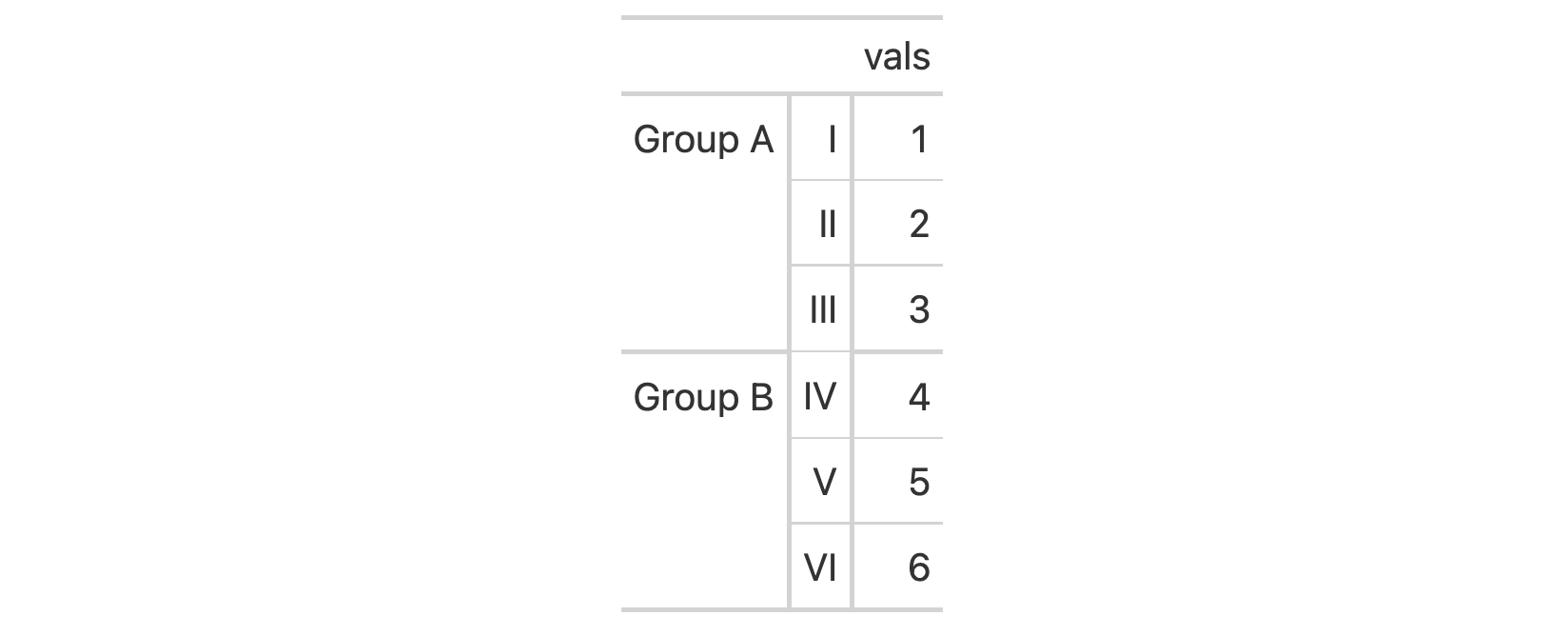Should you need to target only the stub column for formatting or other operations, the stub() select helper can be used. This obviates the need to use the name of the column that was selected as the stub column.

## Usage

stub()

## Value

A character vector of class "stub_column".

## Examples

Create a tibble that has a row column (values from 1 to 6), a group column, and a vals column (containing the same values as in row).

tbl <-
dplyr::tibble(
row = 1:6,
group = c(rep("Group A", 3), rep("Group B", 3)),
vals = 1:6
)

Create a gt table with a two-column stub (incorporating the row and group columns in that). Format the row labels of the stub with fmt_roman() to obtain Roman numerals in the stub; we're selecting the stub column here with the stub() select helper.

tbl %>%
gt(rowname_col = "row", groupname_col = "group") %>%
fmt_roman(columns = stub()) %>%
tab_options(row_group.as_column = TRUE)## Function ID

7-5

Other helper functions: adjust_luminance(), cell_borders(), cell_fill(), cell_text(), cells_body(), cells_column_labels(), cells_column_spanners(), cells_footnotes(), cells_grand_summary(), cells_row_groups(), cells_source_notes(), cells_stub_grand_summary(), cells_stub_summary(), cells_stubhead(), cells_stub(), cells_summary(), cells_title(), currency(), default_fonts(), escape_latex(), google_font(), gt_latex_dependencies(), html(), md(), pct(), px(), random_id()